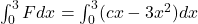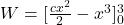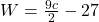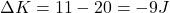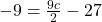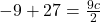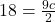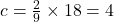## A force Fx=cx-3.00×2 acts on a virus as the virus moves along an x axis, with F measured in Newtons, x in meters, and c a constant. At x = 0

Question

A force Fx=cx-3.00×2 acts on a virus as the virus moves along an x axis, with F measured in Newtons, x in meters, and c a constant. At x = 0, the virus’s kinetic energy is 20.0 J, while at x = 3.00 m, it is 11.0 J. Find c.

in progress 0
6 months 2021-08-14T23:46:49+00:00 1 Answers 2 views 0

4

Explanation:

We are given that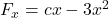K.E at x=0 m=20 J

K.E at x=3 m=11 J

We have to find the value of c.

By work energy theorem

Work done=Change in kinetic energy

W=